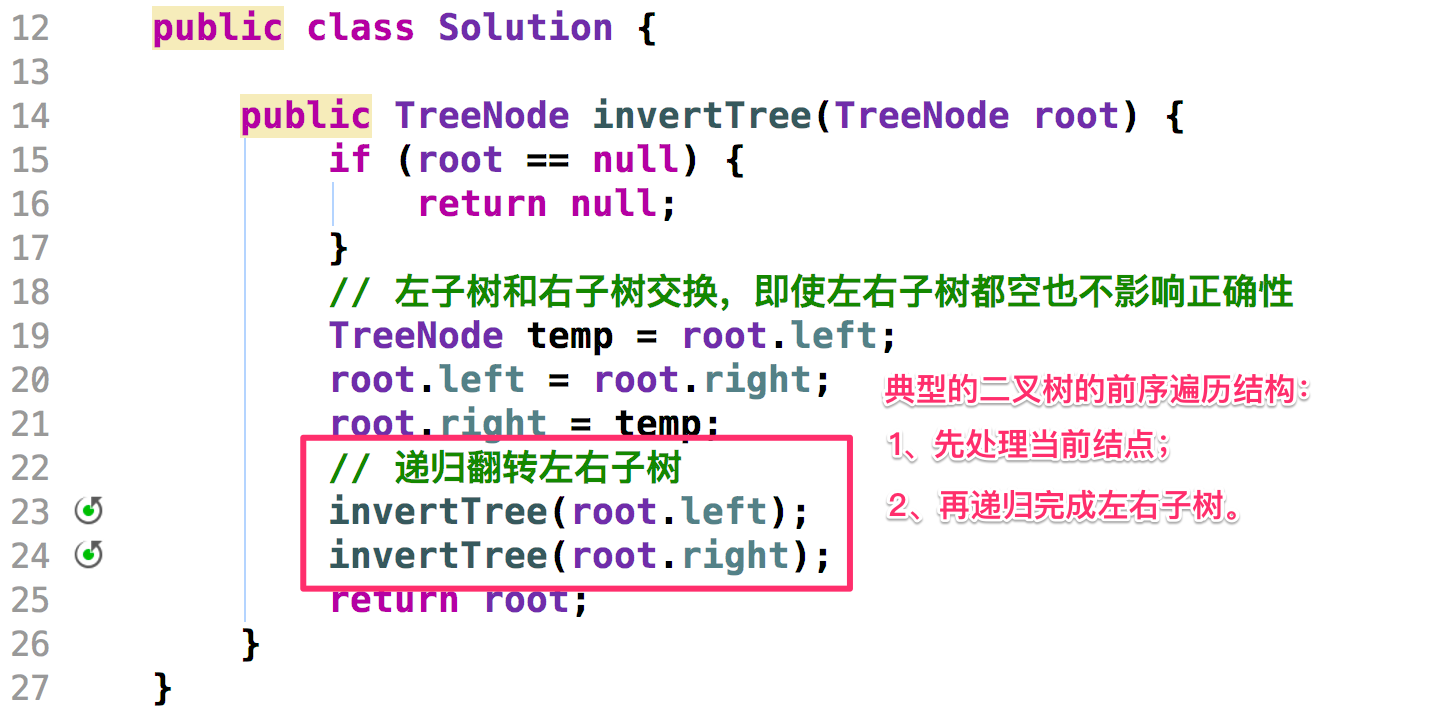# 226. Invert Binary Tree

## 思路分析## 参考解答

### 参考解答1

``````
class TreeNode {
int val;
TreeNode left;
TreeNode right;

TreeNode(int x) {
val = x;
}
}

public class Solution {

public TreeNode invertTree(TreeNode root) {
if (root == null) {
return null;
}
// 左子树和右子树交换，即使左右子树都空也不影响正确性
TreeNode temp = root.left;
root.left = root.right;
root.right = temp;
// 递归翻转左右子树
invertTree(root.left);
invertTree(root.right);
return root;
}
}
``````

### 参考解答2

``````import java.util.LinkedList;

public class Solution2 {

public TreeNode invertTree(TreeNode root) {
// 结点为空的特殊情况要先考虑
if (root == null) {
return null;
}
while (!queue.isEmpty()) {
TreeNode curNode = queue.removeFirst();
// 只要其中之一非空，我都交换，并且把非空的添加到队列里
if (curNode.left != null || curNode.right != null) {
// 先翻转
TreeNode temp = curNode.left;
curNode.left = curNode.right;
curNode.right = temp;
// 把非空的节点加入队列
if (curNode.left != null) {